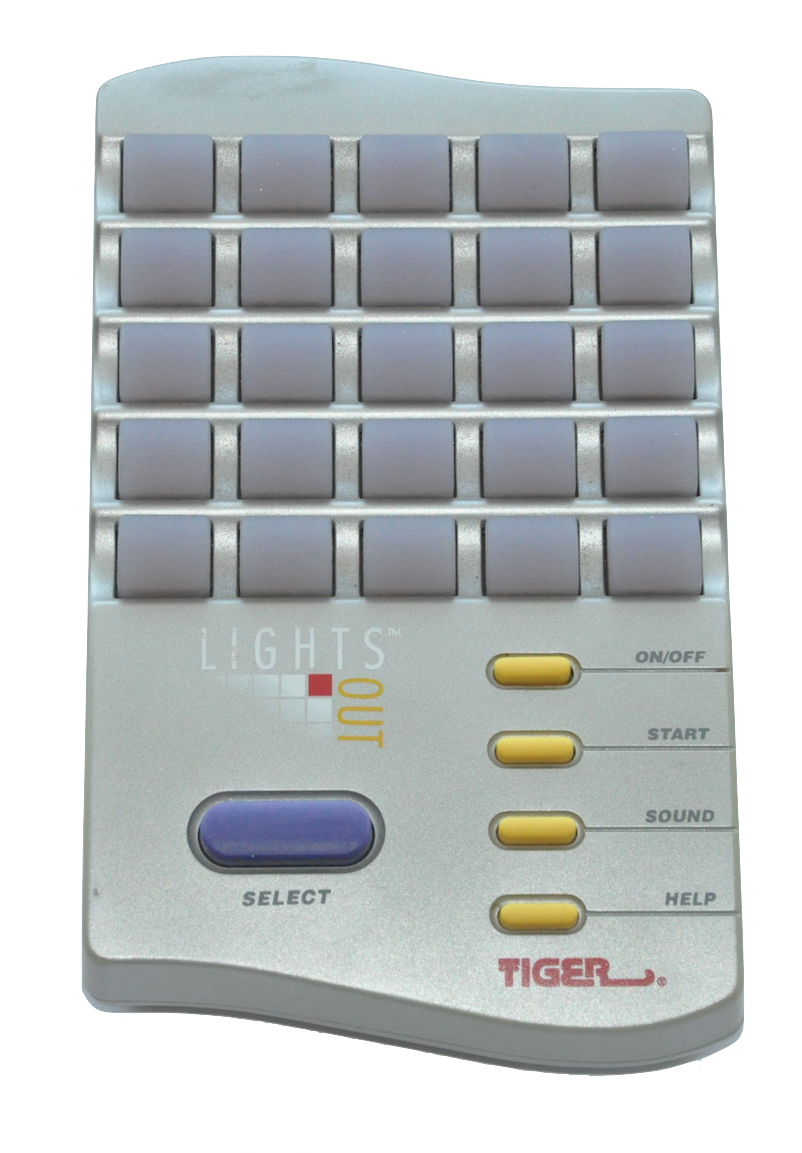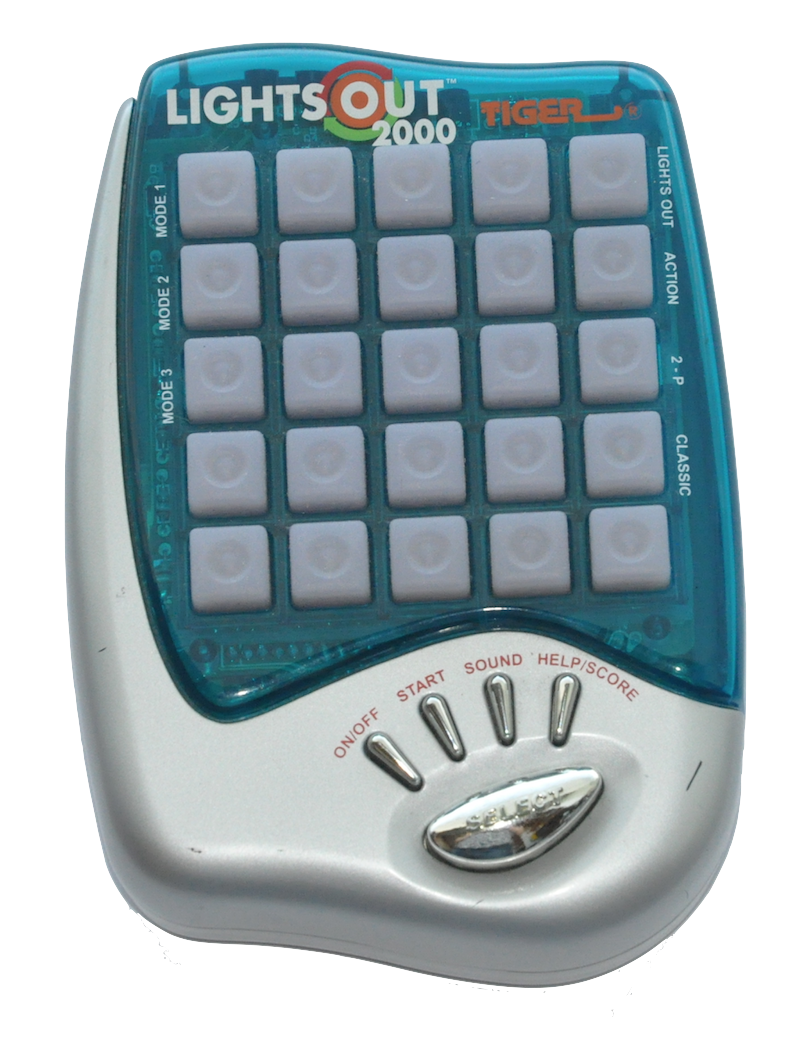## Lights OutThis electronic puzzle consists of a 5-by-5 grid of lights; when the game starts, a set of these lights (random, or one of a set of stored puzzle patterns) are switched on. Pressing one of the lights will toggle it, and the four lights adjacent to it, on and off. The game provides a puzzle: given some initial configuration where some lights are on and some are off, the goal is to switch all the lights off, preferably in as few button presses as possible.

The applet below was written by Jaap Scherphuis © ( Jaap's Puzzle Page).

### Lights Out Matrix

In Lecture 24 of the course notes we show that: writing $\boldsymbol{b}=(b_{1,1}, b_{1,2},b_{1,3}, \ldots, b_{4,5}, b_{5,5})$ for the $25$ dimensional vector representing the light configuration (entries are $1$ if the light at position $(i,j)$ is on, $0$ if off), a strategy vector $\boldsymbol{x}$ for solving the puzzle is a solution to matrix equation (over the finite field $\mathbb{F}_2$) $$A\boldsymbol{x} =\boldsymbol{b}$$ where $A$ is the $25\times 25$ matrix whose columns are the toggle vectors $\boldsymbol{t}_{k,\ell}=(t_{1,1}, t_{1,2}, \ldots, t_{4,5}, t_{5,5})$ (entry $(i,j)$ is $1$ if pressing button $(k,\ell)$ toggles light $(i,j)$, or $0$ otherwise): $$A=\left[\boldsymbol{t}_{1,1}~|~\boldsymbol{t}_{1,2}~|~\cdots ~|~ \boldsymbol{t}_{5,5} \right].$$ We can write $A$ as $$A= \begin{bmatrix} C & I_5 & 0 & 0 & 0 \\ I_5 & C & I_5 & 0 & 0 \\ 0 & I_5 & C & I_5 & 0 \\ 0 & 0 & I_5 & C & I_5 \\ 0 & 0 & 0 & I_5 & C \end{bmatrix} \quad\quad \text{ (lights out matrix)}$$ where $C$ represents the $5\times 5$ matrix $$C= \begin{bmatrix} 1 & 1 & 0 & 0 & 0 \\ 1 & 1 & 1 & 0 & 0 \\ 0 & 1 & 1 & 1 & 0 \\ 0 & 0 & 1 & 1 & 1 \\ 0 & 0 & 0 & 1 & 1 \end{bmatrix}$$ and $I_5$ denotes the $5\times 5$ identity matrix. The matrix $A$ is referred to as the lights out matrix. Using the command block_matrix() we can input $A$ into SageMath as follows.

The above implementation of $A$ is fine for analyzing the $5\times5$ puzzle but we'd like to do a general analysis for different sized game boards. In this case it will be useful for us to implement $A$ as a function which takes the size of the board as input. We can do this as follows.

An alternative construction for $A$ is given below (this is also the one found in the course textbook). You can use either one, just try to undertand the construction in either case.

### Lights Out Solver

Here is an implementation of a lights solver. Scroll to the bottom of the code block for an example.

### Lights Out Variation - 3 states of lights

A variation of the game in which each light has $3$ states (red, green, off) was marketed as Lights Out 2000. The toggle criteria is the same: pressing a button toggles the state of the button pressed and its horizontal and vertical neighbours. The lights cycle through the states red to green to off.

You can play around with this version here. This applet was written by Jaap Scherphuis © ( Jaap's Puzzle Page).

### Lights Out Variation - Different Toggle Patterns and Board Sizes

Jaaps Puzzle Page (lights out) contains tons of information about variations of the puzzle. Try this applet (Lights Out Variations) which allows you to change the board size and toggle patterns. You can try out a cross pattern (x) instead of the classic plus pattern (+).